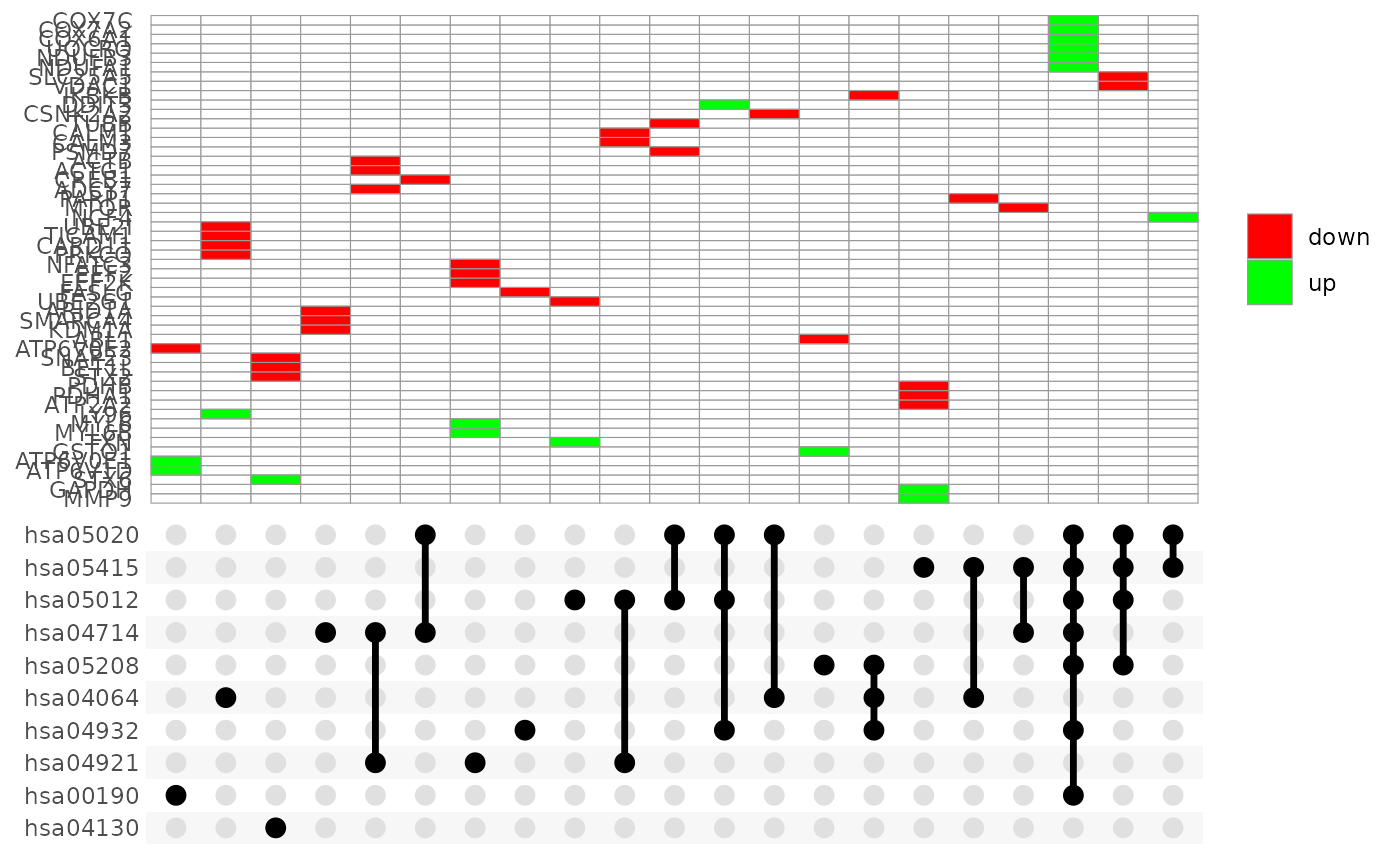Create UpSet Plot of Enriched Terms

UpSet_plot(
result_df,
genes_df,
num_terms = 10,
method = "heatmap",
use_description = FALSE,
low = "red",
mid = "black",
high = "green",
...
)

## Arguments

result_df

A dataframe of pathfindR results that must contain the following columns:

Term_Description

Description of the enriched term (necessary if use_description = TRUE)

ID

ID of the enriched term (necessary if use_description = FALSE)

lowest_p

the highest adjusted-p value of the given term over all iterations

Up_regulated

the up-regulated genes in the input involved in the given term's gene set, comma-separated

Down_regulated

the down-regulated genes in the input involved in the given term's gene set, comma-separated

genes_df

the input data that was used with run_pathfindR. It must be a data frame with 3 columns:

1. Gene Symbol (Gene Symbol)

2. Change value, e.g. log(fold change) (optional)

3. p value, e.g. adjusted p value associated with differential expression

The change values in this data frame are used to color the affected genes

num_terms

Number of top enriched terms to use while creating the plot. Set to NULL to use all enriched terms (default = 10)

method

the option for producing the plot. Options include 'heatmap', 'boxplot' and 'barplot'. (default = 'heatmap')

use_description

Boolean argument to indicate whether term descriptions (in the 'Term_Description' column) should be used. (default = FALSE)

low

a string indicating the color of 'low' values in the coloring gradient (default = 'green')

mid

a string indicating the color of 'mid' values in the coloring gradient (default = 'black')

high

a string indicating the color of 'high' values in the coloring gradient (default = 'red')

...

additional arguments for input_processing (used if genes_df is provided)

## Value

UpSet plots are plots of the intersections of sets as a matrix. This function creates a ggplot object of an UpSet plot where the x-axis is the UpSet plot of intersections of enriched terms. By default (i.e. method = 'heatmap') the main plot is a heatmap of genes at the corresponding intersections, colored by up/down regulation (if genes_df is provided, colored by change values). If method = 'barplot', the main plot is bar plots of the number of genes at the corresponding intersections. Finally, if method = 'boxplot' and if genes_df is provided, then the main plot displays the boxplots of change values of the genes at the corresponding intersections.

## Examples

UpSet_plot(example_pathfindR_output)